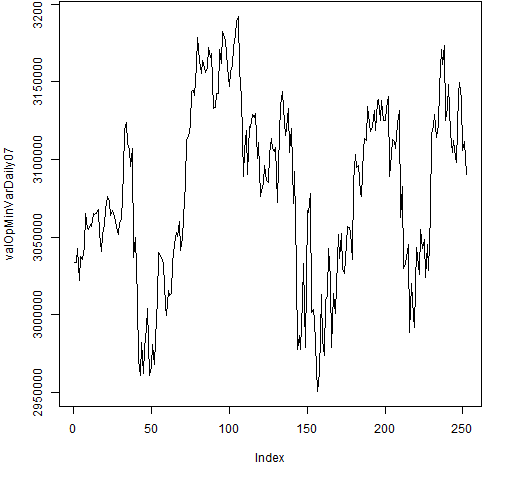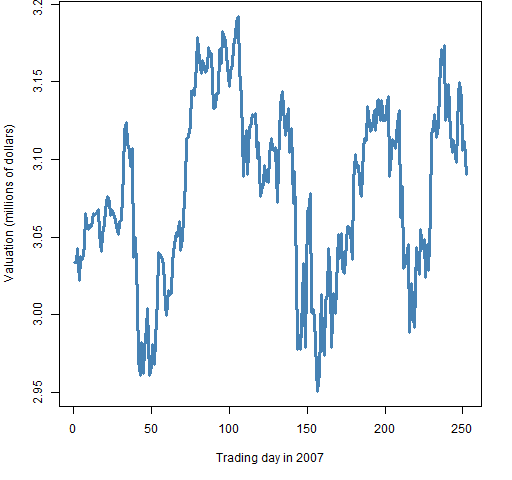## Create and plot portfolio valuations

Compute the valuation of a  portfolio at one or more times.  These can then be plotted.

## Preparation

This presumes that you have or can create a portfolio object.  For example, that you have mastered “Passive, no benchmark (minimum variance)”.

• portfolio object
• Portfolio Probe

You need a portfolio object with which to create valuations.

You also need to have the Portfolio Probe package loaded into your R session:

`require(PortfolioProbe)`

If you don’t have Portfolio Probe, see “Demo or Buy”.

### Doing the example

`require(pprobeData)`

## Doing it

• the valuation at one point in time
• the valuation path through time

### Valuation at one point in time

We create the valuation by using prices at the time point of interest:

```valOpMinVarEnd07 <- valuation(opMinVar,
xassetPrices[502,], collapse=TRUE)```

This uses the prices at the close of the last trading day of 2007, so one year after the portfolio was formed.

The valuation is:

```> format(valOpMinVarEnd07, nsmall=2, big.mark=",")
prices
"3,090,027.16"```

### Valuation path through time

Instead of focusing on one specific time, we look at the evolution of value over time.

To do this we need to use a matrix of prices for the times (and assets) of interest:

```valOpMinVarDaily07 <- valuation(opMinVar,
xassetPrices[251:502,], collapse=TRUE)```

The result is a vector with a value for each time in the price matrix that we gave to `valuation`.

We can just plot the path without trauma:

` plot(valOpMinVarDaily07, type="l")`

The result of that command is Figure 1.

Figure 1: Default plotting of valuation path.A more pleasing plot can be produced using something like:

```plot(valOpMinVarDaily07/1e6, xlab="Trading day in 2007",
ylab="Valuation (millions of dollars)", , type="l",
col="steelblue", lwd=3)```

The command above produces Figure 2.

Figure 2: More pleasing plot of the valuation path.## Explanation

The `valuation` function can do quite a lot.  We’ve seen that it can take prices at either one time or multiple times and return something sensible.

The `collapse` argument controls the granularity of the result.  When it is `FALSE`, then you get information on the individual assets.  When it is `TRUE`, you get information on the portfolio as a whole.  (It is possible to have something in between as well.)

## Further details

We have given the `valuation` function an object that was the result of a call to `trade.optimizer`.  But we didn’t need to.  We would get the same thing if we gave it a vector that described the portfolio — that is, a vector of the number of units of each asset where the names are the asset identifiers.

In particular, these commands give us the same answer:

```valOpt <- valuation(opMinVar, xassetPrices[500:503,],
collapse=TRUE)
valVec <- valuation(opMinVar\$new.portfolio,
xassetPrices[500:503,], collapse=TRUE)```

We compare the two return vectors by binding them into a matrix, and show the vector that we give to `valuation` in the second instance:

```> rbind(valOpt, valVec)
2007-12-27 2007-12-28 2007-12-31
valOpt    3105810    3111558    3090027
valVec    3105810    3111558    3090027
2008-01-02
valOpt    3059643
valVec    3059643
> opMinVar\$new.portfolio
XA105 XA280 XA298 XA643 XA675 XA709 XA731 XA778
2659 17920  2906 20192  7182  6485  4166  6840
XA891 XA966
5200  6247```